## Questions1. Given triangle ABC with vertices A (-6, 5), B(-4, 1) and C(3, 2) and that A(-6, 5) is mapped onto A1(-6, -4) by a shear with y-axis in variant. On the grid provided below;
1. draw triangle ABC
2. draw triangle A1B1C1, the image of triangle ABC, under the shear
3. determine the matrix representing the shear
2. Triangle A1B1C1 is mapped onto A11B11C11 by a transformation defined by the matrix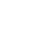1. Draw triangle A11B11C11 on the same grid as ABC and A1B1C1
2. Describe fully a single transformation that maps A11B11C11
1.
1. Under a certain rotation A( 2,0) is mapped onto A1(-4, 2) and B(0,5) is mapped onto B1(-9, 0)
1. On the grid provided plot the lines AB and A1B1 on the same axes
2. Hence determine by construction the co-ordinates of the centre and angle of rotation
2. Under a quarter positive turn about the origin O, A1 is mapped onto A11 and B1 is mapped onto B11. Determine the co-ordinates of A11 and B11
3. Describe fully a single transformation which would map A to A11 and B to B11
2. A transformation T is represented by the matrix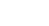and transformation U by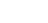the matrix. Given that a rectangle has co-ordinates at A (1,2) B(6, 2), C(6, 4) and D (1, 4) and that under T the image of ABCD is A1B1C1D1 and under U the image of A1B1C1D1 is A2B2C2D2:
1. Find the co-ordinates of A1B1C1D1 and A2B2C2D2
2. On the grid provided, plot ABCD, A1B1C1D1 and A2B2C2D2
3. Describe the transformation represented by:-
1. U
2.  UT
4. If A2B2C2D2 were to be transformed by a transformation represented by the matrix to map onto A3B3C3D3 . What would be the area of A3B3C3D3
3. The vertices of a quadrilateral are A(2,2) B(8,2), C (8,6) and D(6,4) under a rotation the images of vertices A and D are A(0,8) and D1(-2, 12).
1. On the grid provided and using the same axes draw the quadrilateral ABCD and the points A1 and D1
2. Determine the centre and angle of rotation
3. Locate the points B1 and C1under the rotation and complete the quadrilateral
4. A translation maps the point P(5, -3) onto P1(2, -5)
1. Determine the translation vector T
2. A Point R1 is the image of R(-2, -3) under the same translation in (a) above, find the magnitude of P1R1
5. Triangle ABC has vertices at A(0, -1), B(4, 3)and C(2,2).
1. Find the coordinates of image triangle A1B1C1 of triangle ABC under translation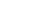2. Given that triangle A11B11C11 is the image of triangle A1B1C1 under an enlargement scale factor 3, centre O(0,0) , find the coordinates of A11, B11and C11
3. If the area of triangle A1B1C1 is 24 cm2, calculate the area of triangle A11B11C11
4. Find the matrix that maps triangle A11B11C11 onto triangle ABC
6.
1. The triangle ABC where A (2,-1) B (1, 2) and C (4, 4) is reflected in the line X = 4 to give triangle A1B1C1. Draw the two triangles on the graph provided and state the co-ordinates of A1B1C1
2. Draw the triangle A2 (5,6), B2 (2,7) and C2 (0,4). Given that triangle A2B2C2 is the image of triangle A1B1C1 under rotation, determine the centre and angle of this rotation
3. Show the image of triangle A2B2C2, under an enlargement centre (0, 6) scale factor -1
7.
1. Find the co-ordinates for the image of point P(6, -2) under the transformation defined by :-
x1= x – 3y
y1= 2x
1. A quadrilateral ABCD has vertices A(4, -3), B(2, -3), C(4, -1) and D(5, -4). On the grid provided, draw the quadrilateral ABCD
2. A1B1C1D1 is the image of ABCD under a rotation through +90o about the origin. On the same axes, draw A1B1C1Dunder the transformation
2. A2B2C2D2is the image of under A1B1C1D1 under another transformation by the matrix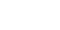1. Determine the co-ordinates of A2B2C2D2and plot it on the same axes
2. Describe the transformation that maps A1B1C1Donto A2B2C2D2
3. Find a single matrix of transformation that would map A2B2C2Donto ABCD
8.
1. Triangle XYZ has vertices X(2, -1) Y(4, -1) and Z (4,2). Triangle XYZ maps onto triangle X1Y1Z1 under transformation T1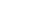. Draw triangles XYZ and its image X1Y1Z1 on the grid provided
2.  Another triangle X11Y11Z11 is the image of X1Y1Z1 after transformation T2
Draw triangle X11Y11Z11 on the same set of axes
3. Find the single transformation matrix T that maps triangle XYZ on to the final image X11Y11Z11
4. Given that the area of triangle XYZ is 15cm2, find the area of the triangle X11Y11Z11
9. The quadrilateral A (2,1), B (4,1), C (4,4) and D (2,4) is mapped onto A’B’C’D’ by a matrix M1 such that A1(8,7), B1(14,7), C1(14,16) and D1(8,16) .
1. Draw both ABCD and A1B1C1D1 on the same plane
2. Find the matrix of transformation that mapped ABCD onto A’B’C’D’ and describe it fully
3. A1B1C1D1 underwent another matrix transformation at N which is a translation that gave the image A11B11C11D11, Where A11(7,9), B11(13,9), C11(13,18) and D11(7,18). The transformation N is a translation . Find the translation
4. Draw A11B11C11D11 on the same axes where ABCD and A1B1 C1D1 were drawn
10.
1. On the grid provided. Plot the points A(2, -1) B (0, -3) C(2, -4) and D (4, -2) and join them to form a quadrilateral ABCD. What is the name of this quadrilateral?
2. The points A1(1, 2) B1(3, 0) C1(4, 2) and D1(2, 4) are the images of ABC and D under a certain transformation T1. On the same grid draw quadrilateral A1B1C1D1 and describe transformation T1 fully.
3. The points A11(-2, -4) B11(-6, 0) C11(-8, -4) and D11(-4, -8) are the images of A1B1C1D1under transformation T2. On the same grid draw quadrilateral A11B11C11D11 and describe the transformation T2 fully.
4. On the same grid draw quadrilateral A111B111C111D111, the image of A11B11C11D11 under a reflection in the x-axis. State the co-ordinates of A111B111C111D111.
11. The Points A1B1 and C1 are the images of A(4, 1), B( 0, -2) and C( -2, 4) respectively under a transformation represented by the matrix;
M=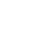1. Write down the coordinates of A1B1 and C1
2. A11B11 and C11 are the images of A1B1 and C1under another transformation whose matrix is:
N =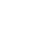Write down the coordinates of A11B11 and C11
3. Transformation M followed by N can be represented by a single transformation P.
Determine the matrix for
4. A matrix P is given by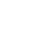Find P-1
12. Triangle A1B1C1 is the image of triangle ABC under a transformation represented by matrix T =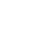If the area of triangle A1B1C1is 25.6cm2, find the area of the object
13. A point P(2, -4) is mapped into P1(4, 0) under a translation.
Determine the image of point Q(-1, 2) under the same translation
14. The points A (2, 6), B (1, 1), C (2, 3) and D (4,0) are the vertices of quadrilateral ABCD.
1. On graph paper plot the points A, B, C, and D and join them to form quadrilateral ABCD.
2. The points A, B, C and D are the images of A1, B1, C1 and D1 respectively under an enlargement centre the origin and scale factor -2. On the same grid draw the image quadrilateral A1B1C1D1.
3. The points A11B11C11 and D11 are the images of ABCD respectively under reflection in the x – axis. On the same grid, locate the pints A11B11C11 and D11 and draw the second image quadrilateral A11B11C11D11.
4. Quadrilateral A111B111C111D111 is the image of ABCD under a certain transformation T.
Describe transformation T fully.
15. T is a transformation represented by the matrix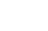. Under T, a square of area 10cm2 is mapped onto a square 110cm2. Find the values of x1.
1.
1. B (4,-5), C (3,6 ½ )
â ABC drawn
â ABC drawn
2. Shear maps
I (1, 1½ )
Matrix = 1  0
1  1½
2.
1.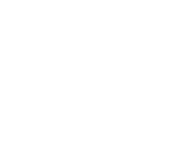2.
1. Centre (-2, -2) 90o
2. A11 (-2 , -4) , B11 (0, 9)
3. Half-turn about the centre (0, 2)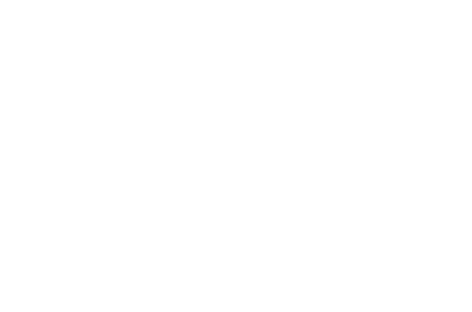3.
1.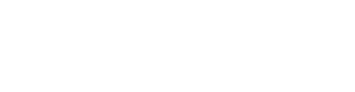2.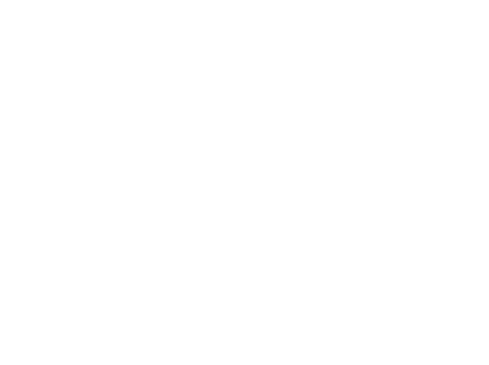1. U - - positive three-quarter turn about the origin
2. UT – Reflection I the line x = 0
3. IdetI = I2.5 x -2 – 1x 0 I= 5
∴ Area = 5x(5x2) = 20sq. units
4.
1.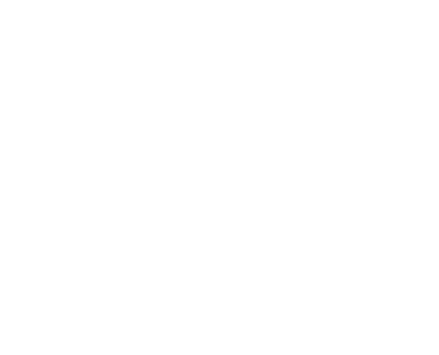2. Centre (-2, 4)
Angle +90°
5.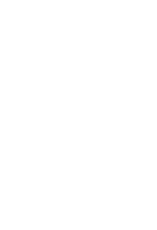6. A1= (0+1, -1-2) = (1, -3)
B
1= (4 + 1) , 3-2) = (4, 1)
C
1 = ( 2 +1, 2-2) = (3-0)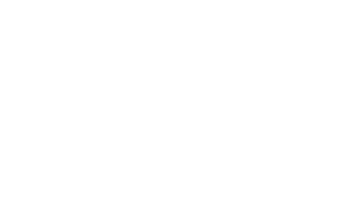7. Scale used S1
ΔABC drawn B1
ΔA1B1C1 drawn B1
A(6, -1), B(7, 2), C(4, 4) B1
Line x = 4L
1
ΔA2 B2 C2 drawn B1
Two seen B1
Centre of rotation
Angle of centre of rotation B
1
A3B3C3 drawn B1

Scale used S
1
ΔABC drawn B1
ΔA1B1C1drawn B1
A, (6, -1), B(7, 2), C(4, 4) B1
Line x = 4 L
1
ΔA2B2C2drawn B1
Two seen B1
Centre of rotation
Angle of centre of rotation B
1
ΔA3B3C3 drawn B1
8.
1. P(6, -2)
X
1 = 6 -3 (-2) = 12
Y
1= 2(6) = 12
(X
1, Y1) = (12, 12)
1. A1(3, 4)
2. B1 (3, 2)
C
1 (1, 4)
D
1(4, 3)
2.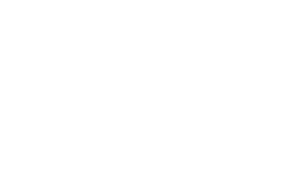9.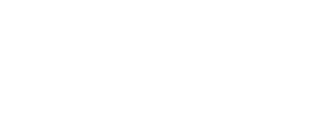10.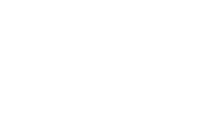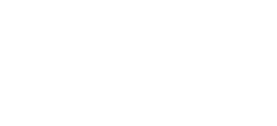11.
1. ABCD drawn B1
Name – Parallelogram B1
2. A1B1C1D1drawn B1
Attempt to joining any two points and bisecting. B1
Description – Rotation + 900. B1 or quarter turn about (0,0)
3. A11B11C11D11 drawn. B1
Description – Enlargement centre (0, 0) Scale factor –Z. B1
4. A111B111C111D111 – drawn. B1
Attempt to reflect. B1
Coordinates
A
111 = 9-2, 4) C111 = (-8, 4) B1All correct
B
111 = (-6, 0) D111 (-4, 8)
12.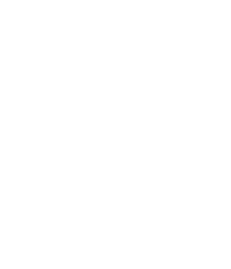13. Det = 2 – 6
= - 4
A.S.F = 4
25.6 = 4
x
x = 6.4cm
2
Area of ΔABC = 6.4cm2
14. T + (2) = (4)
-4       0
T = (4)  - (2) = (2)
(0)    (+4)     (4)
(2) + (-1) = (1)
(4)    (2)     (6)
Q (1,6)
15. 5x2 + 6 = 110/10
5x2 + 6 = 11
x
2= 1
x = ±
1